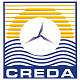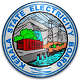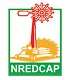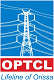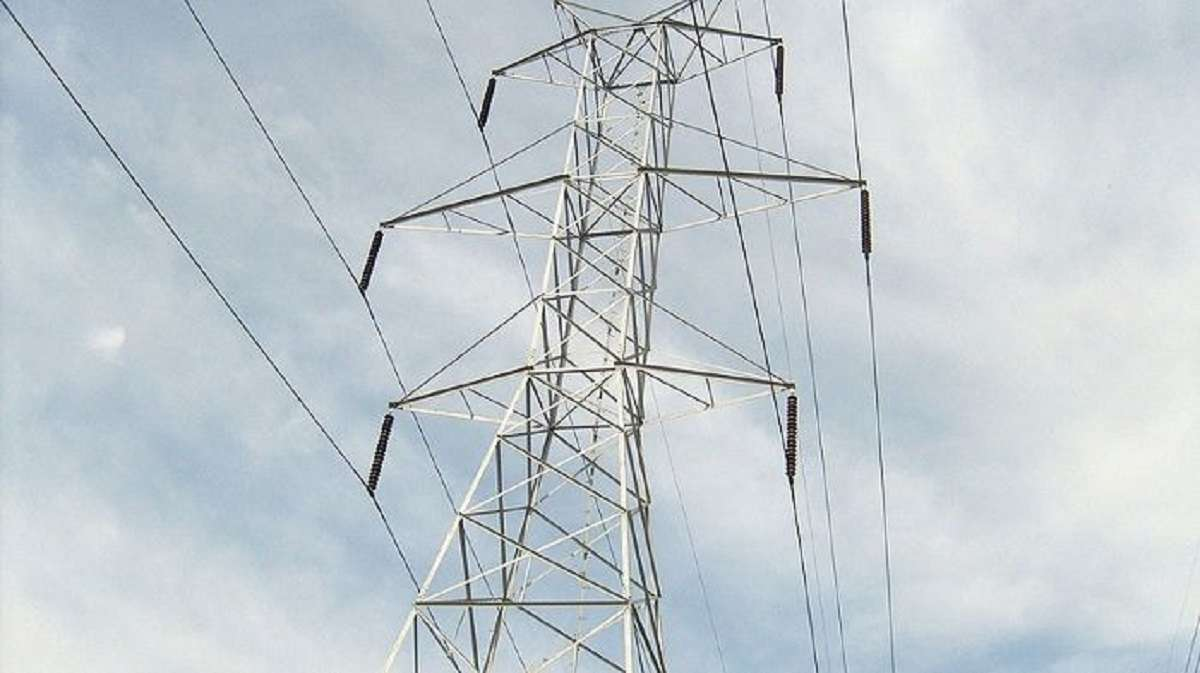# Transmission Line

A transmission line is used for the transmission of electrical power from generating substation to the various distribution units. It transmits the wave of voltage and current from one end to another. The transmission line is made up of a conductor having a uniform cross-section along the line. Air act as an insulating or dielectric medium between the conductors.

For safety purpose, the distance between the line and ground is much more. The electrical tower is used for supporting the conductors of the transmission line.Tower are made up of steel for providing high strength to the conductor. For transmitting high voltage, over long distance high voltage direct current is used in the transmission line.##### Parameters of transmission line

The performance of transmission line depends on the parameters of the line. The transmission line has mainly four parameters, resistance, inductance, capacitance and shunt conductance. These parameters are uniformly distributed along the line. Hence, it is also called the distributed parameter of the transmission line.

The inductance and resistance form series impedance whereas the capacitance and conductance form the shunt admittance. Some critical parameters of transmission line are explained below in detail.

Line inductance -The current flow in the transmission line induces the magnetic flux.When the current in the transmission line changes, the magnetic flux also varies due to which emf induces in the circuit. The magnitude of inducing emf depends on the rate of change of flux. Emf produces in the transmission line resist the flow of current in the conductor, and this parameter is known as the inductance of the line.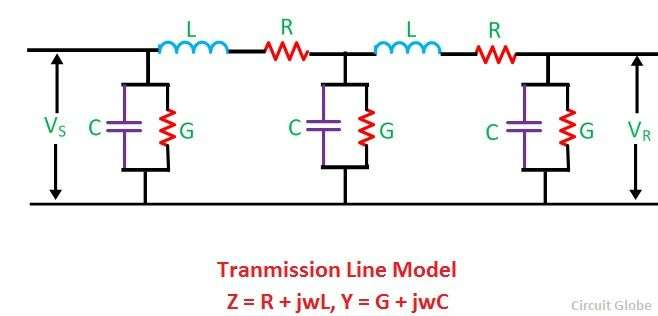Line capacitance – In the transmission lines, air acts as a dielectric medium. This dielectric medium constitutes the capacitor between the conductors, which store the electrical energy, or increase the capacitance of the line. The capacitance of the conductor is defined as the present of charge per unit of potential difference.

Capacitance is negligible in short transmission lines whereas in long transmission; it is the most important parameter. It affects the efficiency, voltage regulation, power factor and stability of the system.

Shunt conductance – Air act as a dielectric medium between the conductors. When the alternating voltage applies in a conductor, some current flow in the dielectric medium because of dielectric imperfections. Such current is called leakage current. Leakage current depends on the atmospheric condition and pollution like moisture and surface deposits.

Shunt conductance is defined as the flow of leakage current between the conductors. It is distributed uniformly along the whole length of the line. The symbol Y represented it, and it is measured in Siemens.

##### Performance of transmission lines

The term performance includes the calculation of sending end voltage, sending end current, sending end power factor, power loss in the lines, efficiency of transmission, regulation and limits of power flows during steady state and transient conditions. Performance calculations are helpful in system planning.

Some critical parameters are explained below

Voltage regulation – Voltage regulation is defined as the change in the magnitude of the voltage between the sending and receiving ends of the transmission line.The efficiency of transmission lines – Efficiency of the transmission lines is defined as the ratio of the input power to the output power.

Some critical parameters are explained below

Voltage regulation – Voltage regulation is defined as the change in the magnitude of the voltage between the sending and receiving ends of the transmission line.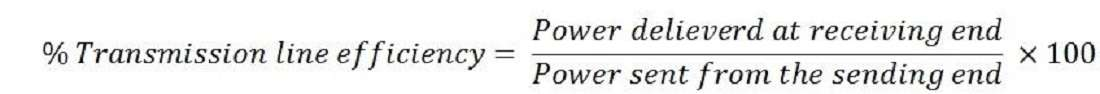##### Important points

- Admittance measures the capability of an electrical circuit or we can say it measures the efficiency of a transmission line, to allows AC to flow through them without any obstruction. It SI unit is Siemens and denoted by the symbol Y.

- Impedance is the inverse of the admittance. Its measure the difficulty occurs in the transmission line when the AC flow. It is measured in ohms and represented by the symbol z.

### Our Clients